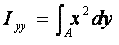Definition and calculation of area moment of inertia or second moment of area of plane section of structural members

Moment of Inertia or second moment of area is a geometrical property of a section of structural member which is required to calculate its resistance to bending and buckling. Mathematically, the moment of inertia of a section can be defined asMoment of Inertia about x-x axisMoment of Inertia about y-y axis

Moment of Inertia of some standard areas can be found below.

1. Rectangular section;

(a)  Ixx = (bd3)/12

(b) Iyy = (db3)/12

where b= width of the section, and d= depth of section.

The axes x-x and y-y are passing through the centroid and x-x axis is parallel to the width of section and y-y parallel to the depth.

2. Circular Section

Ixx = Iyy = (πD4)/64,

where D is the diameter of the section and x-x and y-y axes are passing through the centroid.

Moment of inertia of hollow sections can also be determined by subtracting the moment of inertia of the removed area from the moment of inertia of original area.

We can use parallel axes theorem to find the moment of inertia about an axis parallel to x-x or y-y,

For example if p-q is an axis parallel to x-x and it is at a distance of 'h' from x-x axis. Then the moment of inertia  Ipq  about p-q axis can be determined as given below;

Ipq = Ixx + Ah2

where A= the area of the section

Parallel axes theorem is also used to determine the moment of inertia of built-up sections.

Polar Moment of inertia is required in case of torsion of structural member.  Polar Moment of Inertia is defined as the Moment of inertia bout an axis perpendicular to the plane of the section and can be calculated by applying perpendicular axes theorem which says that

Ixx + Iyy = Izz , where zz-axis is perpendicular to both xx-axis and yy-axis.

Use our Moment of Inertia calculator to determine centroid, moment of inertia, section modulus and radius of gyration for different sections including angle, circle, rectangle, Channel, I or H-section, T-section, pentagon, hexagon. Moment of inertia is required to determine bending stress, shear stress and deflection of beam.

You can select from the list of plane sections given below or visit Instructions for Moment of Inertia Calculator for guidelines on using this calculator

#### Select from the following links to start calculation

Stress Transformation Calculator
Calculate Principal Stress, Maximum shear stress and the their planes

To determine Absolute Max. B.M. due to moving loads.

Bending Moment Calculator
Calculate bending moment & shear force for simply supported beam

Moment of Inertia Calculator
Calculate moment of inertia of plane sections e.g. channel, angle, tee etc.

Reinforced Concrete Calculator
Calculate the strength of Reinforced concrete beam

Moment Distribution Calculator
Solving indeterminate beams

Deflection & Slope Calculator
Calculate deflection and slope of simply supported beam for many load cases

Fixed Beam Calculator
Calculation tool for beanding moment and shear force for Fixed Beam for many load cases

BM & SF Calculator for Cantilever
Calculate SF & BM for Cantilever

Deflection & Slope Calculator for Cantilever
For many load cases of Cantilever

Overhanging beam calculator
For SF & BM of many load cases of overhanging beam

Civil Engineering Quiz
Test your knowledge on different topics of Civil Engineering

Research Papers
Research Papers, Thesis and Dissertation

List of skyscrapers of the world
Containing Tall building worldwide

Forthcoming conferences
Containing List of civil engineering conferences, seminar and workshops

Profile of Civil Engineers
Get to know about distinguished Civil Engineers

Professional Societies
Worldwide Civil Engineers Professional Societies